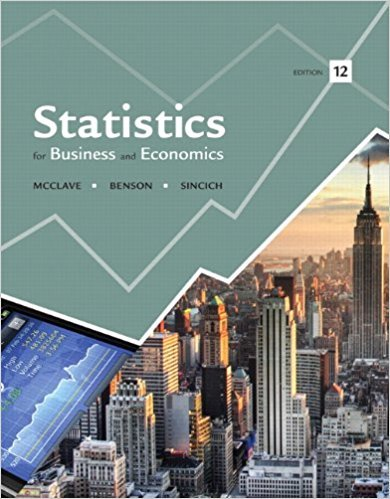×
×

# Suppose the events B1, B2, and B3 are mutually exclusiveISBN: 9780321826237 51

## Solution for problem 78E Chapter 3

Statistics for Business and Economics | 12th Edition

• Textbook Solutions
• 2901 Step-by-step solutions solved by professors and subject experts
• Get 24/7 help from StudySoup virtual teaching assistantsStatistics for Business and Economics | 12th Edition

4 5 0 402 Reviews
24
2
Problem 78E

Suppose the events B1, B2, and B3 are mutually exclusive and complementary events, such that P(B1) = .2, P(B2) = .15, and P(B3) = .65. Consider another event A such that P(A|B1) = .4, P(A|B2) = .25, and P(A|B3) = .6. Use Bayes’s Rule to find

a. P(B1|A)

b. P(B2 |A)

c. P(B3 |A)

Step-by-Step Solution:

Step 1 of 3:

Let the events,, andare mutually exclusive and complementary events.

So,, and.

We consider another,, and.

Our goal is:

a). We need to find.

b). We need to find.

c). We need to find.

a).

Now we use Bayes’s rule we have to find.

First we need to find.=We substituteandvalues.0.8

Therefore,0.8.

Now we need to find.=We substituteandvalues.0.0375

Therefore,0.0375.

Then we need to find.=We substituteandvalues.0.39

Therefore,0.39.

Now we have to find P(A).

P(A) =++We know that,, and.

P(A) = 0.8+0.0375+0.39

P(A) = 0.5075

Therefore, P(A) = 0.5075.

Now we use Bayes’s rule we have to find.

The formula foris=We know thatand.== 0.642

Therefore,= 0.642.

Step 2 of 3:

b).

Now we use Bayes’s rule we have to find.

The formula foris=We know thatand.== 0.073

Therefore,= 0.73.

Step 3 of 3

##### ISBN: 9780321826237

Unlock Textbook Solution